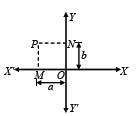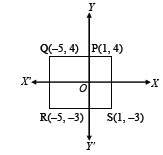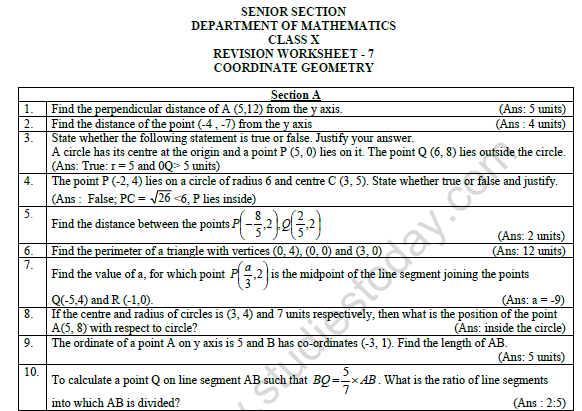# CBSE Class 10 Mathematics Coordinate Geometry Worksheet Set B

Download printable Mathematics Class 10 Worksheets in pdf format, CBSE Class 10 Mathematics Coordinate Geometry Worksheet Set B has been prepared as per the latest syllabus and exam pattern issued by CBSE, NCERT and KVS. Also download free pdf Mathematics Class 10 Assignments and practice them daily to get better marks in tests and exams for Grade 10. Free chapter wise worksheets with answers have been designed by Standard 10 teachers as per latest examination pattern

## Worksheet for Class 10 Mathematics Chapter 7 Coordinate Geometry

Class 10 Mathematics students should refer to the following printable worksheet in Pdf for Chapter 7 Coordinate Geometry in Grade 10. This test paper with questions and solutions for Standard 10 Mathematics will be very useful for tests and exams and help you to score better marks

### Chapter 7 Coordinate Geometry Class 10 Mathematics Worksheet Pdf

CASE STUDY - 1

Rajeev went out from his house to reach the office. But he had to get some work done before going to the office. So, he first of all went to the bank first, from there he went to his son's school, and then reaches to office. The position of home, school, bank and office on coordinate axis is shown in the following figure:(i) If Rajeev goes directly from bank to his office, how much distance he would travel?
(a) 2√97 units
(b) 9√17 units
(c) 7√7 units
(d) 7√45 units

(ii) How much distance he will travel, if goes directly from home to the office?
(a) 15√7 units
(b) 10 units
(c) 14√3 units
(d) 11√5 units

(iii) If at the mid-point of the bank and school, there is a park, what are the coordinates of the park?
(a) (13, 14)
(b) (9,11)
(c) (-5, 10)
(d) (10, 12)

(iv) Find the distance of the point (-6, 8) from the origin.
(a) 8 units
(b) 10 units
(c) 11 units
(d) 9 units

Question. Find the values of y for which the distance between the points P (2, -3) and Q (10, y) is 10 units.
y = 3 or y = -9

Question. If two adjacent vertices of a parallelogram are (3,2) and (-1,0) and the diagonals intersect at (2, -5), then find the coordinates of the other two vertices.
(1,-12) and (5,-10)

Question. If (1, 2), (4, y), (x, 6) and (3, 5) are the vertices of a parallelogram taken in order find x and y.
x = 6 and y = 3

Question. Find the area of a rhombus if its vertices are (3, 0), (4, 5), (-1, 4) and (-2, -1) taken in order.

Question. Find the point on the x-axis which is equidistant from (2, -5) and (- 2, 9).
(-7,0)

Question. Find the ratio in which the line segment joining A (1, – 5) and B (- 4, 5) is divided by the x-axis. Also find the coordinates of the point of division.
k =1 and (-3/2, 0)

Question. Find the coordinates of the points of trisection of the line segment joining (4, -1) and (-2, -3).
(2, -5/3) and (0, -7/3)

Question. Find a relation between x and y such that the point (x, y) is equidistant from the point (3, 6) and (- 3, 4).
Answer : 3x + y - 5 = 0

Question. Find the type of quadrilateral formed by the points (- 1, – 2), (1, 0), (- 1, 2), (- 3, 0) and justify your answer.
Square

Question. Find the centre of the circle passing through A (6, -6), B (3, -7) and C (3, -3).
(4,-5)

Coordinate Geometry

Question. Determine (i) Abscissa (ii) ordinate (iii) Co-ordinates of point P given in the following figure.Answer : (i) Abscissa of the point P = – NP = – OM = – a
(ii) Ordinate of the point P = MP = ON = b
(iii) Co-ordinates of point P = (abscissa, ordinate) = (–a, b)

Question. Draw a triangle ABC where vertices A, B and C are (0, 2), (2, – 2), and (–2, 2) respectively.
Answer : Plot the point A by taking its abscissa O and ordinate = 2.
Similarly, plot points B and C taking abscissa 2 and –2 and ordinates – 2 and 2 respectively. Join A, B and C. This is the required triangle.Question. Draw a rectangle PQRS in which vertices P, Q,R and S are (1, 4), (–5, 4), (–5, –3) and (1, – 3) respectively.
Answer : Plot the point P by taking its abscissa 1 and ordinate – 4.
Similarly, plot the points Q, R and S taking abscissa as –5, –5 and 1 and ordinates as 4, – 3 and –3 respectively.
Join the points PQR and S. PQRS is the required rectangle.